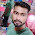### Program 12: To swap two numbers using 3rd variable

Program 12:

```#include<stdio.h>
main()
{
int a,b,c;
printf("Enter a\n");
scanf("%d",&a);
printf("Enter b\n");
scanf("%d",&b);
printf("The values of a is %d and b is %d before swaping\n",a,b);
c=a;
a=b;
b=c;
printf("The values of a is %d and b is %d after swaping\n",a,b);
}```
Explanation:
1. Here we initialized a,b,c
• a---> for storing 1st number
• b--->for storing second number
• c---->for storing temporary variable
2. Now here goes the logic(let us take a=1 and b=3)
• c=a, Therefore, c=1
• a=b, Therefore a=3
• b=c, Therefore b=1
• Now the values of a and b are 3 and 1
3. The values of a and are printed.
Output:

#### 1 comment:

1.factorial hundred In the last few days, the “factorial of 100” is one of the top subjects and a lot of maths geeks compute it using voice assistants such as Alexa, Shiri, etc.

factorial hundred In the last few days, the “factorial of 100” is one of the top subjects and a lot of maths geeks compute it using voice assistants such as Alexa, Shiri, etc.

factorial hundred In the last few days, the “factorial of 100” is one of the top subjects and a lot of maths geeks compute it using voice assistants such as Alexa, Shiri, etc.

 Donate Buy me a coffee \$2.00 USD Buy me a burger \$5.00 USD Buy me a pizza \$10.00 USDCompiler Used by me C-Free 5.0(Recommended) Other Compilers Code Blocks(Recommended) Online Compilers Ideone(Remember to give input before executing online where ever necessary)